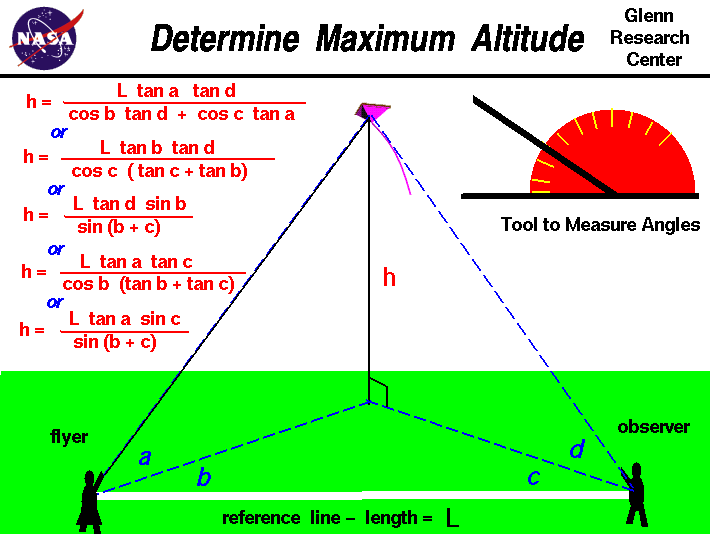+ Text Only Site
+ Non-Flash Version
+ Contact GlennAn excellent way for students to gain a feel for aerodynamic forces is to fly a kite. Students can also use math techniques learned in high school to determine the altitude of the kite during the flight. On this page we show a simple way to determine the altitude of a flying kite. The procedure requires an observer in addition to the kite flyer, and a tool, like the one shown in the upper portion of the figure, to measure angles. The observer is stationed some distance L from the flyer along a reference line which is shown in white on the figure. You can lay a string of known length along the ground between the flyer and the observer to make this reference line. A long line will produce more accurate results. To determine the altitude, the flyer calls out "Take Data", and measures the angle a between the ground and the location of the kite. This measurement is taken perpendicular to the ground. The flyer then measures the angle b between the kite and the reference line. This measurement is taken parallel to the ground and can be done by the observer facing the kite, holding position, and measuring from the direction the observer is facing to the reference line on the ground. When the observer hears the call, "Take Data", the observer must face the kite and measure the angle d from the ground to the kite. The observer must then measure the angle c, parallel to the ground, between the direction the observer is facing and the reference line in the same manner as the flyer. Angles a and d are measured in a plane that is perpendicular to the ground while angles b and c are measured in a plane parallel to the ground. With the four measured angles and the measured distance between the observers, we can use some relations from trigonometry to derive an equation for the altitude h of the rocket. The equation is h = (L * tan a * tan d) / ( cos b * tan d + cos c * tan a) where the tangent (tan) and the cosine (cos) are trigonometric functions whose values are determined using tables or a calculator. A detailed analysis of this trigonometry problem indicates that we really only need three angle measurements along with the reference length measurement to completely determine the answer. The angles a, b, c, and d are related to each other and we can eliminate one of the angle measurements and still determine the altitude. h = (L * tan b * tan d) / (cos c * (tan c + tan b)) An alternative equation, which is equivalent to the previous equation, is also shown on the figure: h = (L * tan d * sin b) / sin(b + c) where the sine (sin) is another trigonometric function. Notice that in this equation you have to add the angles b and c before evaluating the sine in the denominator. This is called a "double angle" formula. If we eliminate angle d, the resulting equation is: h = (L * tan a * tan c) / (cos b * (tan b + tan c)) An alternative equation, which is equivalent to the previous equation, is also shown on the figure: h = (L * tan a * sin c) / sin(b + c) You can use any of these equations to determine the height of any object from a tall tree to a flying rocket. If you have your flyer and observer take all four angle measurements, you can actually make three calculations of the height, which can help to eliminate errors in the measurments. If you do not know trigonometry, you can still determine the altitude of the kite by using a graphical solution from the four angle measurements. As a further check, here's a Java calculator which will solve the equations presented on this page. This page shows an interactive Java calculator which solves the equations for the measured altitude of a kite in flight. You can enter the four angles shown in the graphic in the white boxes labeled "Angle A" through "Angle D". You then choose the mode for the calculation using the drop down menu. Mode #1 uses all four measured angles, Mode #2 ignores angle a, and Mode #3 ignores angle d. You perform the calculations by pushing the red "Compute" button. You should compare all three calculated results, your own hand calculations, and a graphical calculation to determine and minimize errors in the measurements. Calculations and input can be entered in either English or Metric units by using the "Units" choice button. You can download your own copy of this calculator for use off line. The program is provided as Altcalc.zip. You must save this file on your hard drive and "Extract" the necessary files from Altcalc.zip. Click on "Altcalc.html" to launch your browser and load the program. Activities: Guided ToursKite FlyingNavigation ..Beginner's Guide Home Page+ Inspector General Hotline + Equal Employment Opportunity Data Posted Pursuant to the No Fear Act + Budgets, Strategic Plans and Accountability Reports + Freedom of Information Act + The President's Management Agenda + NASA Privacy Statement, Disclaimer, and Accessibility CertificationEditor: Nancy Hall NASA Official: Nancy Hall Last Updated: May 13 2021 + Contact Glenn